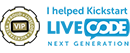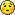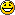## List of combinations

Got a LiveCode personal license? Are you a beginner, hobbyist or educator that's new to LiveCode? This forum is the place to go for help getting started. Welcome!

Moderators: Klaus, FourthWorld, heatherlaine, kevinmiller

MaxV
Posts: 1574
Joined: Tue May 28, 2013 2:20 pm
Location: Italy
Contact:

### List of combinations

Hello,
I need to list all combinations, how can I do it?
For example: numbers 1 to 10, list all combinations of 3 numbers:
1,2,3
2,3,4
3,5,6
1,3,4
... and so on.
The formula to get how many combinations are is:in this example is: (10*9*8*7*6*5*4*3*2*1)/((7*6*5*4*3*2*1)*(3*2*1)) = 3628800 / 30240 = 120; so I know that the example list is made of 120 lines.
How can I list all lines?
Livecode Wiki: http://livecode.wikia.com
My blog: https://livecode-blogger.blogspot.com
To post code use this: http://tinyurl.com/ogp6d5w

sritcp
Posts: 368
Joined: Tue Jun 05, 2012 5:38 pm
Location: Alexandria, Virginia

### Re: List of combinations

My suggestion would be to

i) get the permutations first (straightforward using repeat loops); then

ii) get the combinations by removing the ordered variants of the same combination.

E.g., we have k! permutations for the combination 1,2,3; namely,
1,2,3
1,3,2
2,1,3
2,3,1
3,1,2
3,2,1
It should be easy to identify and remove them.

Regards,
Sri

[-hh]
VIP Livecode Opensource BackerPosts: 2069
Joined: Thu Feb 28, 2013 11:52 pm
Location: Göttingen, DE

### Re: List of combinations

Code: Select all

``````on mouseUp
put 0 into nb
repeat with x=1 to 10
repeat with y=x+1 to 10
repeat with z=y+1 to 10
add 1 to nb -- nb is just for info
put cr & nb & ": " & (x,y,z) after s
end repeat
end repeat
end repeat
put line 2 to -1 of s into fld 1
end mouseUp``````
Here are 3 elements taken out of 10.
This is also a listing for n=10 and k=7, the listing is then the 3 elements that are not taken.
In general combinations(n,k) = combinations(n,n-k)

For recursive building of such lists you could use the logic of Pascal's triangle:
combinations(n-1,k) + combinations(n-1,k-1) = combinations(n,k) for 0 < k < n.
shiftLock happens

dunbarx
VIP Livecode Opensource BackerPosts: 6068
Joined: Wed May 06, 2009 2:28 pm
Location: New York, NY

### Re: List of combinations

Here is a function from the masterLibrary:

Code: Select all

``````function combinatorial n
put the cursor into tCursor
put 1 into tCursorCtr
if n >= 15 then -- make sure you know what you're in for!
answer n && "objects will result in" && (2^15 -1) && "subsets" with "Continue" or "Cancel"
if it is "Cancel" then exit to top
end if
put 1 into subsetsVar -- initialize variable
repeat until x = n -- this repeats (2^n - 2) times, which is one less than the numer of possible subsets
Add 1 to tCursorCtr
if tCursorCtr > 5000 then
set the cursor to busy
put 0 into tCursorCtr
end if
put the last line in subsetsVar into x -- each subset is used as input to compute the next subset
put the number of items in x into noItems
if item noItems of x = n then
add 1 to item (noItems -1) of x -- increment 2nd to last item if last item = n
delete the last item of x -- get rid of last item if it = n
put return & x after subsetsVar -- add another subset to list of subsets
else
put ((item noItems of x) + 1) into item (noItems + 1) of x -- increment last item
put return & x after subsetsVar -- add another subset to list of subsets
end if
end repeat
set the cursor to tCursor
return subsetsVar -- return the list of all possible subsets
end combinatorial``````
Try running this with, say, "4".Once you get the output, you can associate each integer with an item in your list of values. You can then sort that list by length, and filter out all but those results that contain three (or any number) of terms.

Craig

MaxV
Posts: 1574
Joined: Tue May 28, 2013 2:20 pm
Location: Italy
Contact:

### Re: List of combinations

dunbarx wrote:Here is a function from the masterLibrary:
Dunbarx,
what is the masterLibrary?
Livecode Wiki: http://livecode.wikia.com
My blog: https://livecode-blogger.blogspot.com
To post code use this: http://tinyurl.com/ogp6d5w

dunbarx
VIP Livecode Opensource BackerPosts: 6068
Joined: Wed May 06, 2009 2:28 pm
Location: New York, NY

### Re: List of combinations

MaxV
Posts: 1574
Joined: Tue May 28, 2013 2:20 pm
Location: Italy
Contact:

### Re: List of combinations

Thank you so much dunbarx, however [-hh] solution is faster (but I needed to search for internet to understand it).

However now I have more questions:
1. how can I transform it in a function like combinations(n,k)?
2. this repeat loops can be paralleled, because it doesn't need the result of the previous cycle, so how can I parallelize it?
Livecode Wiki: http://livecode.wikia.com
My blog: https://livecode-blogger.blogspot.com
To post code use this: http://tinyurl.com/ogp6d5w

dunbarx
VIP Livecode Opensource BackerPosts: 6068
Joined: Wed May 06, 2009 2:28 pm
Location: New York, NY

### Re: List of combinations

Hermann.

Help Max. You are the one to do it.

Craig

[-hh]
VIP Livecode Opensource BackerPosts: 2069
Joined: Thu Feb 28, 2013 11:52 pm
Location: Göttingen, DE

### Re: List of combinations

Factorials are 'exploding' numbers. So, if n is not too large, say n <= 21, and k <= n/2,
using numOfComb(n,k) = numOfComb(n,n-k),
the following recursion runs pretty fast. It's a "generalization" of the (n=10,k=3) example above.

Code: Select all

``````-- usage examples: get combin(n,k) or get combin(42,4)
-- ordering of the ouput is lexicographical
function combin n,k,r,j,c
if r is empty then put 1 into r --> r admits only nums above the current
if j is empty then put 1 into j --> j counts from 1 up to k
repeat with i=r to n
put i into item j of c
-- we now reduce to the "rest": the nums > i and <=n
if j < k then put combin(n,k,i+1,j+1,c) after s0
else put cr & c after s0
end repeat
return s0
end combin``````
Here is a code for testing speed. If n > 42 or (n > 21 and k > 4), the result is not written to a field but to a file.
Timing is for the algorithm only, not for field- or file-writing.

Code: Select all

``````on mouseUp
set the recursionLimit to 50000000
put the millisecs into m1
lock screen; lock messages
set cursor to watch
put 42 into n; put 4 into k ## <--------------
if k < 1 or k > n then exit mouseUp
if n > 42 or (n > 21 and k > 4) then
put the effective filename of this stack into f
set itemdel to slash
put "out(" &k& "of" &n& ").txt" into last item of f
set itemdel to comma
end if
put the millisecs into m1
put combin(n,k) into cc -- result has a leading cr
put (-1+the num of lines of cc) into n0
put the millisecs - m1 into m2
put the internet date &cr& \
m2 & " ms for " & n0 & " combinations " & \
"(" & k & " out of " & n & ")" into nfo
if not (n > 42 or (n > 21 and k > 4)) then put nfo & cc into fld 1
else
put nfo &cr& "written to " & f into fld 1
put n0 & cc into url("file:"&f)
end if
unlock screen; unlock messages
end mouseUp``````
Once again the differences over the LiveCode version are interesting.
The output string for 4_of_100 = 3921225 combinations has 45.8 MByte.

LC 6.7.11:    6 seconds for 4_of_100 [comparison base]
LC 7.1.4:    21 seconds for 4_of_100 [factor 3.5 compared to LC 6]
LC 8.1.3:    21 seconds for 4_of_100 [factor 3.5 compared to LC 6]
LC 9.0.0:    33 seconds for 4_of_100 [factor 5.5 compared to LC 6]

Absolute times are from a medium fast machine (Mac mini, 2.5 GHz), rounded down, roughly.
I don't know the reason for these extreme differences, which are not necessarily present for some other examples.

== Edit. Adjusted the "write to field-/write to file-switch".
Last edited by [-hh] on Wed Jan 25, 2017 8:28 am, edited 1 time in total.
shiftLock happens

[-hh]
VIP Livecode Opensource BackerPosts: 2069
Joined: Thu Feb 28, 2013 11:52 pm
Location: Göttingen, DE

### Re: List of combinations

Anticipating your next question:
It may be also interesting to look at an "inductive law n--> n+1" for that.

For the number of combinations we have (visualized by Pascal's triangle)
 numOfCombs(n,k-1) + numOfCombs(n,k) = numOfCombs(n+1,k) for 0 < k <= n.

This is an "inductive law n->n+1", i.e. if you have computed the number of combinations for a fixed n and all k <= n, then you can get the number of combinations for all k <= n+1 from that.
Recall the case k-1=0 is the one possibility to take no element out of n.

For the combinations we have similarly an "inductive law n->n+1":
 combin*(n,k-1) &cr& combin(n,k) = combin(n+1,k) for 0 < k <= n.

Here combin*(n,k-1) is built as follows:
Take combin(n,k), without the leading cr, and put comma & n+1 after each line of it.

Recall the case k-1=0 is the one combination to take no element out of n, an empty line.

That is, if you have computed the combinations for a fixed n and all k <= n, then you can get the combinations for all k <= n+1 from that.
But the result of this induction is no longer sorted lexicographically. One way to solve this is to sort it after concatenating:

Code: Select all

`````` -- lexicographic ordering of lines with k items
repeat with j=k down to 1
sort rslt numeric by item j of each
end repeat
``````
The formula  above is intuitively clear:
You have everything computed for a fixed n and now the new element n+1 appears.
This changes the combinations for taking k out of n+1 as follows.
First we have combin(n,k), that is the combinations of taking k and n+1 is not included, what remains unchanged.
Then we have additionally the combinations of taking k and n+1 is included.
That is, writing it out sorted, take k-1 out of the first n and then additionally n+1, that is the list combin*(n,k-1) as described above.

This "inductive law" allows (theoretically, using 'string'-addition) to compute the combination lists for _any_ n (depending on hardware limits only), while the recursive formula has a platform dependent limit for n from the recursion depth.

[But remember the huge sizes of the files.
Using decimal representation in bytes combin(100,4) has 3921225 lines and length 45799908 Bytes, combin(100,3) has 161700 combinations and length 1416492 Bytes,
so combin(101,4) has 3921225+161700=4082925 combinations and length 45799908+1416492+4*161700 = 47863200 Bytes]
shiftLock happens

[-hh]
VIP Livecode Opensource BackerPosts: 2069
Joined: Thu Feb 28, 2013 11:52 pm
Location: Göttingen, DE

### Re: List of combinations

I was asked to script the "inductive law" for building lists of combinations.
So here it is.
The inductive start (n=1) is in the buildcombiBib script below.
The inductive step "n -> n+1":

Code: Select all

``````-- in mouseUp set the local variables f0 and l0:
-- put the effective filename of this stack into f0
-- put "%03d" into l0 -- length 3, leading zeros: for outfile()
on inductiveCombi i -- step "i-1 -> i"
repeat with j = 1 to i-1
put url ("file:" &  outfile(j-1,i-1)) into s1 -- k=j-1, n=i-1
put url ("file:" &  outfile(  j,i-1)) into s2 -- k=j  , n=i-1
put empty into s3
repeat for each line L in s1
if L is empty then put cr & i after s3
else put cr & L & comma & i after s3
end repeat
put s2 & s3 into s4
set itemdel to comma
repeat with m = j down to 1
sort s4 numeric by item m of each
end repeat
put s4 into url ("file:" & outfile(j,i)) -- k=j, n=i
end repeat
-- k=0 and k=i for n=i
put cr into url("file:" & outfile(0,i))
put url ("file:" & outfile(i-1,i-1)) &comma& i \
into url("file:" & outfile(i,i))
end inductiveCombi

function outfile k,n
put f0 into f1
set itemdel to slash
put "out(" &format(l0,k)& "of" &format(l0,n)& ").txt" into last item of f1
set itemdel to comma
return f1
end outfile``````
You can build with that "inductively" files of lists of combinations(n,k)
for increasing n and all k with 0 <= k <= n, starting from n=1.

Code: Select all

``````-- in mouseUp set the local variables f0 and l0:
-- put the effective filename of this stack into f0
-- put "%03d" into l0 -- length 3, leading zeros: for outfile()
on buildcombiBib n2
## n2 = 10 needs 50 millisecs for 32 KByte data (65 files)
## n2 = 16 needs 500 millisecs for 2.9 MByte data (152 files)
## n2 = 21 needs 18 seconds for 107 MByte data (252 files)
## n2 = 24 needs 3 minutes for 1 GByte data (325 files)
put the millisecs into m1
lock screen; lock messages
set cursor to watch
-- inductive start -- i=1
put cr  into url ("file:"& outfile(0,1)) -- k=0, i=1
put "1" into url ("file:"& outfile(1,1)) -- k=1, i=1
-- inductive build -- repeatedly step "i-1 -> i"
repeat with i = 2 to n2
inductiveCombi i
end repeat
put n2 & ": " & (the millisecs - m1) into fld "out2"
unlock screen; unlock messages
end buildcombiBib``````
A button script example for building a "bib" for n from 1 up to a value n2
or building another single step on top of such a "bib" is here.

Code: Select all

``````local f0, l0

on mouseUp
put the effective filename of this stack into f0
put "%03d" into l0 -- length 3, leading zeros: for outfile()
buildcombiBib 16 -- needs 500 ms
put the millisecs into m1
inductiveCombi 16 -- last step on top of the 15-bib needs 150 ms
put cr & "n=16: " & the millisecs -m1 after fld "out2"
put the millisecs into m1
inductiveCombi 17 -- another step on top of the 16-bib needs 350 ms
put cr & "n=17: " & the millisecs -m1 after fld "out2"
end mouseUp
``````
Timing denoted in the scripts is from a Mac min 2.5 GHz using **LC 6.7.11** on MacOS 10.12.

I wrote most of it just out of my head, tested a few times, compared some to "direct" (recursive) computations, couldn't find an error.

Now have fun!
shiftLock happens

dunbarx
VIP Livecode Opensource BackerPosts: 6068
Joined: Wed May 06, 2009 2:28 pm
Location: New York, NY

### Re: List of combinations

I wrote most of it just out of my head
That's our Hermann.FourthWorld
VIP Livecode Opensource BackerPosts: 6713
Joined: Sat Apr 08, 2006 7:05 am
Location: Los Angeles
Contact:

### Re: List of combinations

Why have file I/O inside the loop?
Richard Gaskin
Community volunteer LiveCode Community Liaison

LiveCode development, training, and consulting services: Fourth World Systems: http://FourthWorld.com
LiveCode User Group on Facebook : http://FaceBook.com/groups/LiveCodeUsers/

SparkOut
Posts: 1983
Joined: Sun Sep 23, 2007 4:58 pm

### Re: List of combinations

[-hh]
VIP Livecode Opensource BackerPosts: 2069
Joined: Thu Feb 28, 2013 11:52 pm
Location: Göttingen, DE

### Re: List of combinations

@FourthWorld
FourthWorld wrote:Why have file I/O inside the loop?
When computing combin(n,k) for k=ceil(n/2) you are, roughly, doubling the size of combin(n-1,k) for k=ceil((n-1)/2).

combin(19,10) has size 2.3 Mbyte
combin(20,10) has size 4.7 MByte
combin(21,11) has size 10.0 MByte
combin(22,11) has size 20.1 MByte

Around (n=32,k=16) you will have crossed 1 GByte size for combin(n,k).
And combin(33,17) is built using combin(32,17) and combin(32,16) ...
And combin(42,21) alone may go close to the limit of your hardware resources (has 538257874440 lines of roughly length 100 each).

If you go high enough, this I/O must be even splitted into parts of files inside the repeat. And other 'compressed' methods of data storing (not decimal bytewise but bitwise) should come in.

Of course the scripts above can/should be optimized regarding I/O, depending on (n,k).
You are the one for that!
Would be an application of several threads where you gave effective receipts for i/o of big data sizes.

@SparkOut
SparkOut wrote:Fascinating reading here: http://newsletters.livecode.com/march/i ... etter4.php
Thanks for that link, one of the famous articles of this author. The linked one is for *permutations* (math term) and the word 'combination' there is not using the math term, rather means 'combining/concatenating letters'.
For *combinations* (math term), what the OP wants, you have to take only such permutations that obey one _fixed_ sort order (for example numeric ascending).
For this math term see https://en.wikipedia.org/wiki/Combination .

===
There are a lot of routines for that problem in the folks, in other languages. And a lot of people are asking for pseudo-code to understand optimized algorithms. That's why I tried to make our own thing in LiveCode:
We can write the code in such a way that it's understandable even for non-LiveCoders, it is (nearly) its own pseudo-code.
shiftLock happens